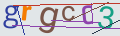# How to calculate SHA256 hash (PHP)

To calculate a hash using SHA256 format for a string in PHP, you need to use the hash function:

``````\$myString = 'test';
\$result = hash('sha256', \$myString);``````

where

\$myString - is the string to be encoded.

The first parameter passed to the hash function is the hashing algorithm, it can be different, for example "md5", "sha1", "haval160,4", etc.

You can find out all available algorithms using the hash_algos() function